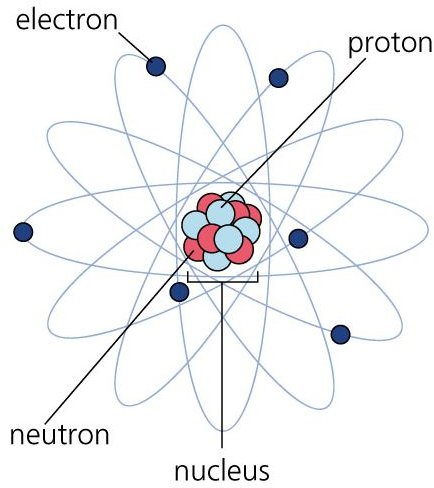# Neil Bohrs atomic model – Learn about the basics of electricity

## Introduction

Electricity is all about the flow of electrons so basically the subatomic level of matter plays an important part in all electrical phenomenons in a subtle manner. Hence it is very important to properly understand the structure of the atom in order to understand electricity. Various models of the atom have been put forward by various scientists and researchers over a period of time. Some of these models were discarded due to anomalies in theory and observation while others have been modified in light of new experiments. We will study one such model which is important from the electrical engineering point of view, and that is that Neil Bohr’s atomic model which we will study in this article.

## Neils Bohr’s Atomic Model

As the name itself suggests, this model of atomic structure was proposed by a scientist named Neils Bohr nearly a century ago, in the year 1913. The main reason for Bohr to put forward this theory just two years after Rutherford has proposed his theory was to explain some serious flaws in the latter. The Bohr’s model was based on the Quantum Theory and the main points of the model are as follows.

• Bohr suggested that the major part of the atomic mass was concentrated at the center of the atom which is known as the nucleus and is positively charged. Of course this was same as Rutherford’s theory. Bohr’s theory also confirmed regarding orbital motion of the electrons around this nucleus.
• Since a rotating body experiences centrifugal force which tries to throw the body outwards, it has to be balanced by some inward force. This is provided by the electrostatic force of attraction between the positively charged nucleus and the negatively charged electrons.
• The main difference between the Bohr’s model vis-à-vis Rutherford’s model is that the former does not allow electrons to revolve in any arbitrary model around the nucleus but only in discrete orbits. The angular momentum of the electrons in these orbits is equal to the integral multiple of a certain constant number. The constant is experimentally calculated as h/2Π where h is known as Planck’s constant.• Because of the fact that the orbits are not arbitrary but discrete, they are known as stationery orbits. They are also known as non-radiating orbits since the theory also states that an electron cannot radiate any energy while being in the same orbit. The adjacent diagram clearly shows the model of the atom in a colourful manner and the various components of the atom are clearly labeled.
• An electron can only emit (or absorb) energy if it jumps from an orbit with higher energy to lower energy (or vice versa). The equation which gives the amount of energy emitted in such a transition is given by the formula dE = h * f where dE is energy change, h is Planck’s constant and f is frequency of emitted radiation.

Of course the above discussion is limited to Bohr’s model which is sufficient for the purposes of basic electricity explanation; yet this theory has several flaws and they have been addressed by other proposals such as the cloud theory. There were models in the past even before Rutherford’s model such as those proposed by Dalton, Thompson etc and there will be models in future also till the mystery of the atom gets resolved completely.

## References

Schneider, J. (2007) Chalkbored. Pace of Mind Publishing